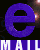Constraints on the chaotic inflationary scenario with a nonminimally coupled inflaton'' field from the cosmic microwave background radiation anisotropy

Eiichiro Komatsu and Toshifumi Futamase
Astronomical Institute, Graduate School of Science, Tohoku University, Sendai 980-8578, Japan

Abstract

We investigate a possibility to restrict the chaotic inflationary scenario with large nonminimally coupled inflaton field $\phi$ considered by Fakir and Unruh by means of the observed cosmic microwave background radiation(CMBR) anisotropy. This model is characterized by the condition $\xi>1$ and $\psi\equiv 8\pi G\xi\phi^2 \gg 1$ where $\xi$ is the nonminimal coupling constant. We calculate the contributions of the long wavelength gravitational waves (GW) generated in the inflationary period to CMBR anisotropy quadrupole moment. We obtain the constraint $\lambda/\xi^2 = 1.8\times 10^{-8}/\psi_{i}$, where $\lambda$ is the self coupling and $\psi_i$ means the initial value of $\psi$. Combining this with previously obtained constraint $\sqrt{\lambda/\xi^2} \approx (\delta T/T)_{\rm rms} = 1.1\times 10^{-5}$, we conclude that the initial value has to be $\psi_i \approx 1.6\times 10^3$. If the self-coupling has a reasonably values of order $10^{-2}$, then $\xi \approx 10^4$ and $\phi_i \approx 10^{-1} m_{pl}$ where $m_{pl}$ is the Planck mass.Please Contact Me !!
Mail: komatsu@astr.tohoku.ac.jp